Learn about smartphone sensors in the next Xcel masterclass with Tinkerbee CEO, Anupam! Learn about smartphone sensors in the next Xcel masterclass with Tinkerbee CEO, Anupam!

# RBSE Maths Class 12 Chapter 11: Application of Integrals: Quadrature Important Questions and Solutions

RBSE Class 12 Maths Chapter 11 – Application of Integrals: Quadrature Important questions and solutions are given here. All the important questions and solutions of this chapter are given here, which help students to score better in their exams. The RBSE Class 12 important questions and solutions provided at BYJU’S have detailed explanations for all the questions.

Chapter 11 of RBSE Class 12 has only two exercises which involve questions on finding the area under a given curve. In this chapter, different types of questions are available such as finding the area enclosed by a parabola, circle, an ellipse and a line. The second exercise has questions on the combination of two curves and the area bounded between them.

### RBSE Maths Chapter 11: Exercise 11.1 Textbook Important Questions and Solutions

Question 1: Find the area enclosed by parabola y2 = 4ax and its latus rectum.

Solution:

Given equation of parabola is:

y2 = 4ax

y = √(4ax) = 2√a √x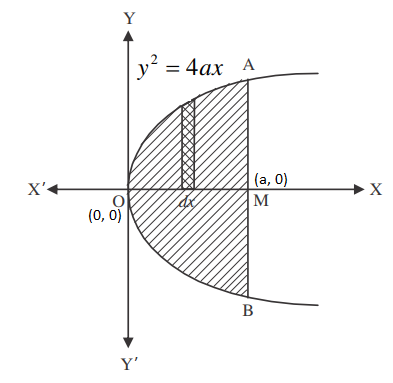Required area = Area of AOBMA

= 2 × Area of AOMA

= 2∫0a y dx

= 2 ∫0a 2 √a √x dx

= 4√a ∫0a (x)1/2 dx

= 4√a [x3/2/ (3/2)]0a

= 4√a × (2/3) × [(a)3/2 – 0]

= (8√a/3) × (a3/2)

= 8a2/3 sq.units

Question 2: Find the area enclosed by line (x/a) – (y/b) = 2 and both axes.

Solution:

Given equation of line is:

(x/a) – (y/b) = 2

(x/a) – 2 = (y/b)

⇒ y = (b/a)x – 2b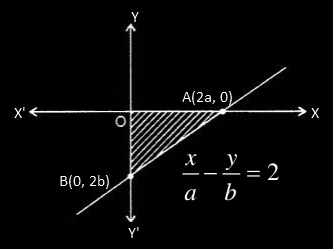Required area = ∫02a y dx

= | ∫02a [(b/a)x – 2b) dx|

= | (b/a) [x2/2]02a – 2b[x]02a|

= |(b/2a) [4a2 – 0] – 2b[2a – 0]|

= |2ab – 4ab|

= |-2ab|

= 2ab sq.units

Question 3: Find the total area of ellipse (x2/a2) + (y2/b2) = 1.

Solution:

Given,

Equation of ellipse is:

(x2/a2) + (y2/b2) = 1

y2/b2 = 1 – (x2/a2)

y2/b2 = (a2 – x2)/a2

y2 = [b2(a2 – x2)]/a2

y = (b/a)√(a2 – x2)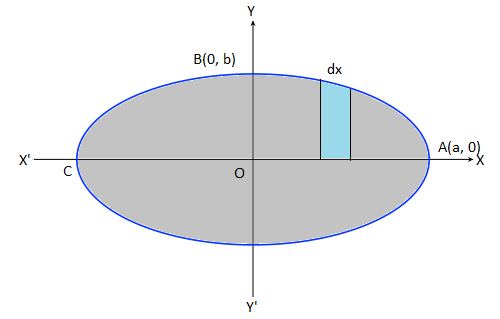Required area = Area of ABCDA

= 4 × Area of AOBA

= 4 × ∫0a y dx

= 4 × ∫0a [(b/a) √(a2 – x2)] dx

= (4b/a) [(1/2) {x√(a2 – x2) – a2 sin-1(x/a)}]0a

= (2b/a) [0 – a2 sin-1(1)]

= -(2b/a) × (πa2/2)

= |-πab|

= πab sq.units

### RBSE Maths Chapter 11: Exercise 11.2 Textbook Important Questions and Solutions

Question 4: Find the area between parabola y2 = 2x and circle x2 + y2 = 8.

Solution:

Given,

Equation of circle is:

x2 + y2 = 8 ….(i)

y2 = 8 – x2

y = √(8 – x2)

Equation of parabola is:

y2 = 2x ….(i)

y = √(2x)

By solving (i) and (ii),

x = -4, 2

x = -4 is not possible for the given equations.

Thus, x = 2

Therefore, the coordinates are:

A(2, 2), D(2, 0), C(2√2, 0)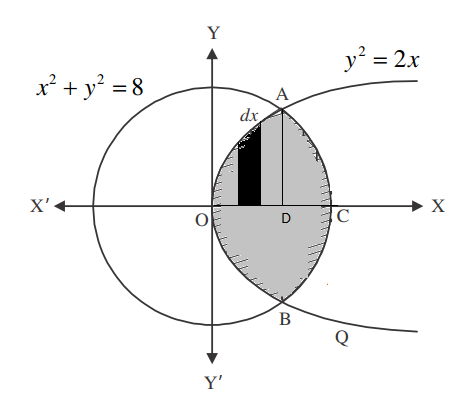Required area = Area of AOBCA

= 2 × AODCA

= 2 [ ∫ y (parabola) dx + ∫ y (circle) dx|

= 2 [ ∫02 √(2x) dx + ∫22√2 √(8 – x2) dx]

= 2 { √2 [x3/2/ (3/2)]02 + [(x/2) √(8 -x2) + (8/2) sin-1(x/2√2)]22√2}

= 2√2 × (2/3) × 23/2 + 2[(2√2/2) √(8 – 8) + 4 sin-1 (2√2/ 2√2) – {(2/2) √(8 – 4) + 4 sin-1(2/2√2)}]

= (16/3) + 8 sin-1(1) – 2 × 2 – 8 sin-1(1/√2)

= (16/3) + 8 × (π/2) – 4 – 8 (π/4)

= (4/3) + 8 × (π/2)

= 2π + (4/3) sq.units

Question 5: Find the area between parabola 4y = 3x2 and line 3x – 2y + 12 = 0.

Solution:

Given,

Equation of parabola is:

4y = 3x2

y = (3x2)/4

Equation of line is:

3x – 2y + 12 = 0

2y = 3x + 12

y = (½)(3x + 12)

The graph of parabola and line intersect each other at (-2, 3) and (4, 12) as shown in the figure below.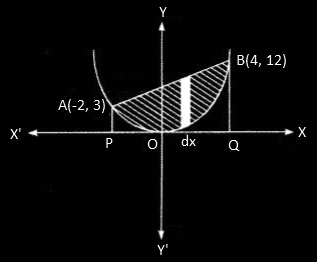Required area = Area of BAOB

= ∫-24 (line) dx – ∫-24 y (parabola) dx

= ∫-24 (1/2)(3x + 12)dx – ∫-24 (3×2/4) dx

= (1/2) × [3x2/2 + 12x]-24 – (3/4) [x3/3]-24

= (3/4) × [16 – 6] + 6[4 + 2] – (1/4) [64 + 8]

= (3/4) × 12 + (6 × 6) – (1/4) × 72

= 9 + 36 – 18

= 27 sq.units

### RBSE Maths Chapter 11: Additional Important Questions and Solutions

Question 1: Find the area bounded by x2 + y2 = 1 and x + y ≥ 1.

Solution:

Given,

Equation of circle is:

x2 + y2 = 1

Equation of line is:

x + y ≥ 1

Let, x + y = 1

y = 1 – x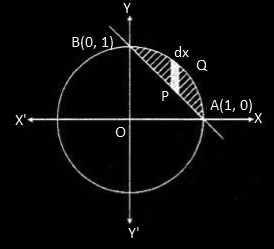Required area = (1/4) × Area of circle – Area of AOBA

= (1/4) × πr2 – Area of AOBA

= (1/4) × π × (1)2 – ∫01 y dx

= (π/4) – ∫01 (1 – x) dx

= (π/4) – [x – (x2/2)]01

= (π/4) – [1 – (1/2)]

= (π/4) – (1/2)

= (π – 2)/4 sq.units

Question 2: Using integration, find the area of a triangle whose vertices are (-1, 0), (1, 3) and (3, 2).

Solution:

Given,

Vertices of a triangle are (-1, 0), (1, 3) and (3, 2).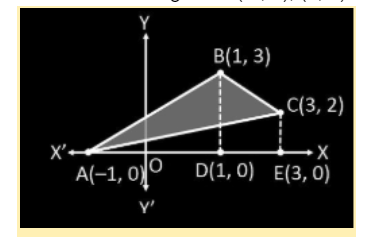Area of triangle ABC = Area of triangle ABD + Area of trapezium BCED – Area of triangle ACE ….(i)

Equation of line AB is:

y – 0 = [(3 – 0)/ 1 – (-1)] [x – (-1)]

y = (3/2) (x + 1) ….(ii)

Equation of line BC is:

y – 3 = [(2 – 3)/ (3 – 1)] (x – 1)

y – 3 = -(1/2) (x – 1)

y = -(1/2)(x – 1) + 3

y = -(1/2)x + (1/2) + 3

y = -(1/2)x + (7/2) ….(iii)

Equation of line AC is:

y – 2 = [(0 – 2)/ (-1 – 3)] (x – 3)

y – 2 = (2/4) (x – 3)

y – 2 = (1/2)(x – 3)

y = (1/2)x – (3/2) + 2

y = (1/2)x + (1/2)

y = (1/2)(x + 1) ….(iv)

Area of triangle ABD = ∫y (line AB) dx

= ∫-11 (3/2)(x + 1) dx

= (3/2) [(x2/2) + x]-11

= (3/2) × [{(1/2) + 1} – {(1/2) – 1}]

= (3/2) × [(3/2) – (-1/2)]

= (3/2) × [(3/2) + (1/2)]

= (3/2) × (4/2)

= 3 sq.units

Area of trapezium BCDE = ∫y (line BC) dx

= ∫13 [(-1/2)x + (7/2)] dx

= ∫13 (7/2) dx – (1/2) ∫13 x dx

= (7/2) ∫13 1.dx – (1/2) ∫13 x dx

= (7/2) [x]13 – (1/2) [(x2)/2]13

= (7/2) × (3 – 1) – (1/2) × [(9/2) – (1/2)]

= (7/2) × 2 – (1/2) × (8/2)

= 7 – 2

= 5 sq.units

Area of triangle ACE = ∫y (line AC) dx

= ∫-13 (1/2)(x + 1) dx

= (1/2) ∫-13 x dx + (1/2) ∫-13 1 dx

= (1/2) [(x2)/2]-13 + (1/2) [x]-13

= (1/2) × [(9/2) – (-1)2/2] + (1/2) × [3 – (-1)]

= (1/2) × [(9/2) – (1/2)] + (1/2) × 4

= (1/2) × (8/2) + 2

= 2 + 2

= 4 sq.units

Substituting all these values in (i),

Area of triangle ABC = 3 + 5 – 4

= 4 sq.units